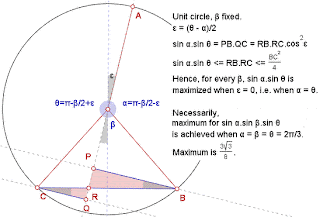## Friday, January 7, 2011

### Fox 319 - Solutions

Below are 2 distinct solutions for Fox 319...
A solution based on symmetry by Bleaug:Trigonometry and calculus by Six:

x + y + z = 360 and sinx*siny*sinz

-> f(x,y) = sin(x)*sin(y)*sin(360-x-y)
-> f(x,y) = sin(x)*sin(y)*-sin(x+y)

Now to find the critical points of f(x,y), we just need to find the partial derivative with respect to x and y and solve for 0.

f_x(x,y) =

sin(x)sin(y)(-cos(x+y)-cos(x)sin(y)sin(x+y)

solve for 0.

sin(x)sin(y)(-cos(x+y)-cos(x)sin(y)sin(x+y)=0

-> tan(x) = -tan(x+y)

Since the function is symmetric, we should get the same partial derivative for y.

-> tan(y) = -tan(y+x)

-> tan(x)=tan(y)

-> x = y or they are opposites. However, if they are opposites, the original function just becomes 0. Thus, x = y.

Now substitute in x for y in the original equation and find its critical points.

Eventually, you will get sin(3x)=0

x = 120 degrees
y = 120 degrees
z = 120 degrees